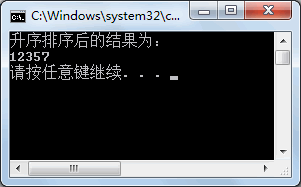## C#冒泡排序（Sort方法）

• 内容
• 评论
• 相关

【实例 1】在 Main 方法中创建一个整型数组，并在该数组中存放 5 个元素，使用冒泡排序算法将数组中的元素从小到大排序。

```class Program
{
static void Main(string[] args)
{
int[] a = { 5, 1, 7, 2, 3 };
for(int i = 0; i < a.Length; i++)
{
for(int j = 0; j < a.Length - i - 1; j++)
{
if (a[j] > a[j + 1])
{
int temp = a[j];
a[j] = a[j + 1];
a[j + 1] = temp;
}
}
}
Console.WriteLine("升序排序后的结果为：");
foreach(int b in a)
{
Console.Write(b + "");
}
Console.WriteLine();
}
}```0条评论